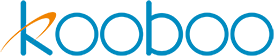zhDocuments

# TypeScript & ES Module

Kooboo supports TypeScript and ES Modules.

ES Modules

ES Modules are JavaScript modules defined by the ECMAScript specification. You can define object methods in one file and reference them in another file.

For example, in the file sample.js, you can define the following:

`````` export function foo(){
return 'bar'
}``````

Then, in another file, you can import and use these functions

``````import {foo} from "./sample"
let text = foo();
k.response.write(text); ``````

TypeScript

In Kooboo, you can directly write TypeScript code in JavaScript files, which will be dynamically compiled into JavaScript during runtime.

You can use types in TypeScript, such as

``````const car: { type: string, model: string, year: number } = {
type: "BYD",
model: "Song",
year: 2021
};``````

Users can define classes in TypeScript as follows

`````` class Person {
private name: string;
public constructor(name: string) {
this.name = name;
}
public getName(): string {
return this.name;
}
}
var newUser = new Person("Smith");
k.response.write(newUser.getName()); ``````

Users can use generics in TypeScript to create reusable components or functions that can work with different data types. Here's an example:

``````class NamedValue<T> {
private _value: T | undefined;

constructor(private name: string) {}

public setValue(value: T) {
this._value = value;
}

public getValue(): T | undefined {
return this._value;
}

public toString(): string {
return `\${this.name}: \${this._value}`;
}
}

let value = new NamedValue<number>('myNumber');
value.setValue(10);

k.response.write(value.toString());``````

Users can define interfaces and implement them in TypeScript.  Here's an example:

``````interface Rectangle {
height: number,
width: number
}

interface ColoredRectangle extends Rectangle {
color: string
}

const coloredRectangle: ColoredRectangle = {
height: 20,
width: 10,
color: "red"
};

k.response.write(coloredRectangle.color); ``````